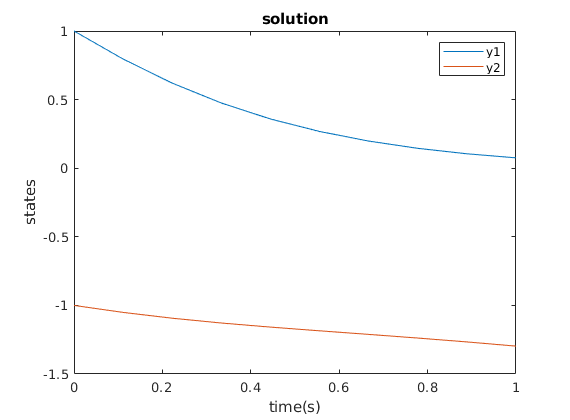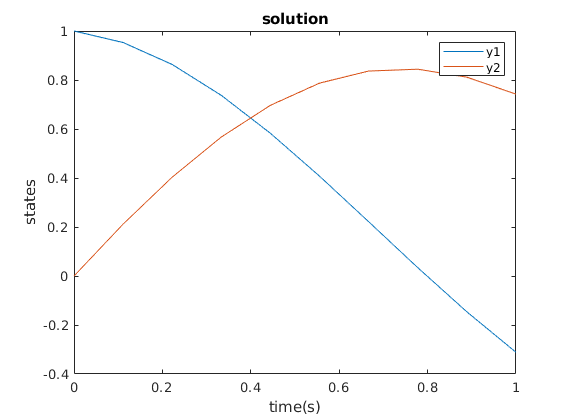# Basic example of dynamics objects

In this example, we present the use of class ‘ode’. Here we solve the following simple system of ordinary differential equations:

In DyCon toolbox, the use of symbolic variables is chosen to represent the dynamic equations. We first define a symbolic vector representing the solution states.

Y = sym('y',[2 1])


Y =

y1
y2



In addition, we define a symbolic vector for the control functions of the equation.

U = sym('u',[2 1])


U =

u1
u2



Using these two variables, we can state the system by a symbolic expression of the vector field.

F = @(t,Y,U,Params) [ sin(Y(1)*Y(2)) +     (Y(1)*Y(2)) + U(1)   ; ...
Y(2)        +  cos(Y(1)*Y(2)) + U(2) ] ;
mu = param('mu',10);
nu = param('nu',10);

parameters = [mu nu];
dynamics = ode(F,Y,U,parameters);


In this way, we defined ‘dynamics’ of the class ‘ode’ which represents the following equation of a matrix form:

Let’s see what we have created

dynamics


dynamics =

ode with properties:

StateVector: [1x1 struct]
Control: [1x1 struct]
DynamicEquation: [1x1 SymNumFun]
Params: [1x2 param]
Derivatives: [1x1 odeDerivatives]
InitialCondition: [2x1 double]
FinalTime: 1
Nt: 10
MassMatrix: [2x2 double]
label: ''
Solver: @eulere
SolverParameters: {}
tspan: [1x10 double]
ControlDimension: 2
StateDimension: 2
dt: 0.1000



DyCon toolbox creates a lot of default variables to represent the conditions of differential equations. We may see the information more heuristic way through the resume function:

resume(dynamics)


Dynamics:

Y'(t,Y,U) = @(t,Y,U,Params)[sin(Y(1)*Y(2))+(Y(1)*Y(2))+U(1);Y(2)+cos(Y(1)*Y(2))+U(2)]

t in [0,1]  with condition: Y(0) = 0 0



We also can directly modify its variables, for example, we may change the initial data:

dynamics.InitialCondition = [1 ; -1];
resume(dynamics)


Dynamics:

Y'(t,Y,U) = @(t,Y,U,Params)[sin(Y(1)*Y(2))+(Y(1)*Y(2))+U(1);Y(2)+cos(Y(1)*Y(2))+U(2)]

t in [0,1]  with condition: Y(0) = 1 -1



‘solve’ function solves the differential equation of ‘ode’. The values of ‘Y’ are calculated according to the timeline and initial data. The MATLAB built-in function ‘ode45’ works as a default to solve the ‘ode’.

solve(dynamics);


‘plot’ function plots the states of ‘Y’, the vector of states. Note that this produces errors if ‘solve’ function is not operated.

plot(dynamics)If the system is linear, then we can create the ‘ode’ class using the matrix. Along with the notion of linear control problem, the following code represents the system:

A = [ 0 -2 ; 2 0];
B = [1 ; 1];

dynamics_linear = ode('A',A,'B',B);
dynamics_linear.InitialCondition = [1;0];
solve(dynamics_linear);
plot(dynamics_linear)